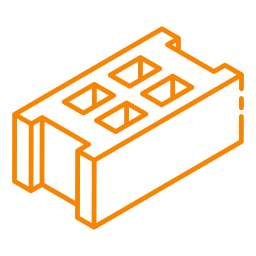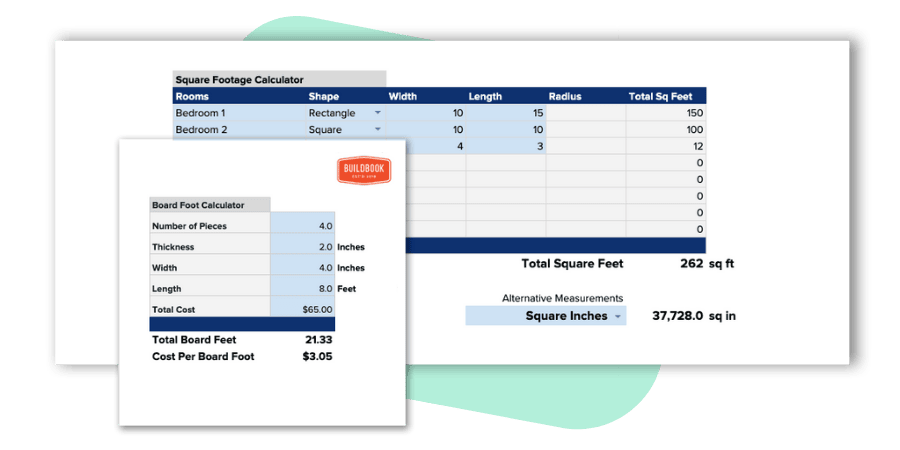# Brick CalculatorCalculate the quantity and total cost of concrete blocks needed based on the dimensions of a wall.

## Looking for an even better free concrete calculator for your construction business?

This collection of construction calculators is 100% free to download, copy, and use as your own. You're free to modify and/or adjust any calculator to best fit the needs of you or your business.This concrete block calculator allows you to estimate how many concrete blocks you'll need based on the block size and width and height of a wall. Additionally, you can determine your total concrete block cost by inputting your cost per block.

## What is the standard size of concrete blocks used in construction?

The standard sizes of concrete blocks used in construction vary depending on the region, but the most common size is 8x8x16 inches. Other sizes include 6x12x12 inches, 8x16x16 inches, and 12x12x12 inches.

## How to calculate the number of concrete blocks you need

To determine the number of concrete blocks you need to construct a wall, you'll need to know two things:

1. The square footage of the wall
2. The size of the concrete block being used

Once you have these to factors determined, the next steps are fairly simple.

### Find the area of a brick wall

Length of wall * height of wall = area of wall

For example, if your wall is 30' wide and 20' long, the square footage of your wall is 600 square feet (30 x 20 = 600). Next we'll find the area of a single concrete block.

### Find the area of a concrete block

Length of block * height of block = area of block

Using a standard 16" x 8" concrete block, we can calculate that the area of a single block is 128 square inches (16 x 8 = 128).

Since the area of the wall was measured in feet and the concrete block was measured in inches, we need to first convert one of them so both areas are based on the same unit of measurement. In this case, we'll convert the area of the concrete block to square feet by dividing 128 / 144 to end up with .889 as the square footage of a single concrete block.

### Find the quantity of concrete blocks

Area of wall / area of block = concrete blocks needed

With the area of both the wall and concrete block determined, we can calculate that we'll need a total of 675 concrete blocks to complete the wall (600 / .889 = 674.9).

If you'd like to find the total cost, simply multiply the total number of concrete blocks by the cost per block (i.e. 675 x \$5.45 = \$3,678.75).

## Do concrete blocks need to be filled?

Concrete blocks do not need to be filled; however, they can be filled with gravel, sand, or a concrete mix to increase their weight and stability. Additionally, concrete blocks can be reinforced with steel rods or mesh for added wall strength.